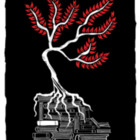0 pointsSubmitted by Walter White

# Can I have a better definition of bitmasks?

I’m making my way through the sections well enough but I don’t quite understand what bitmasks are and what they do.

A mask is simply a name given to a a certain binary number that will let you do various operations on another binary number.

I find it is easiest to understand if you have examples.

The two times you will need a mask are when you want to check a certain bit or change a certain bit.

First, checking a bit.

If I tell you what the 2nd bit from the right is for 1010, you can just look at it and tell me 1. But if I’m writing a program, and I want to have a separate task for if that bit is a 1 or if that bit is a 0, and I don’t know what binary number will be passed through this program, then I need to devise a way to check the bit.

There is no built in function that lets me check bits. So I have to do it myself.

Consider the ways you can check a bit, using logical operators. Specifically, think of AND.

0 & 0 = 0 0 & 1 = 0 1 & 0 = 0 1 & 1 = 1

Look at those bolded ones. If I AND a bit with 1 (a number that I know and set), then I will be returned the value of that bit. If that bit is a 0, 1 AND 0 is 0. If it is a 1, 1 AND 1 is 1.

So the 1 that I set is a mask. I know what it is, and I am using it to perform operations on another binary number.

If I have a longer binary number, and I want to check the 3rd bit from the right, I can make a corresponding mask, I would set my mask to be 000100. I would then AND that mask with the binary number. I would then receive with 000000 or 000100 as my result. So, if the result is equal to 0, then that bit is a 0. Else, it is a 1 (000100 would obviously equal 4, and so the returned value rarely equals exactly 1, so it is easier to check if it is 0 or not).

Here is a modular example that will check any bit in “number”, specified by “n”.

``````def checkbit(number,n):
# The 1 in the mask will be shifted until it reaches the correct spot
if mask & number == 0:      #If that operation returns 0
print "The bit is a 0"
else:
# If that operation returns anything not 0 (1, 2, 4, 8, etc.)
print "The bit is a 1"``````

The other reason for using masks is to manipulate a bit.

If I want to set a certain bit to 1, but I don’t know what that bit is originally, I need to use an operator. Or works here.

0 OR 0 = 0 0 OR 1 = 1 1 OR 0 = 1 1 OR 1 = 1

Again, look at the last two. Assume the 1 on the left is the mask, and the value on the right of the operator is the value I’m checking. No matter what that value is, 0 or 1, if I OR it with 1, the bit will always be 1.

Thus, I will create a mask that is equal to 1 at the bit I would like to change and a 0 everywhere else. When I OR this with the original binary, I will retrieve a result that is the exact same as the original binary, except for the single bit being changed.

pointsSubmitted by Hurricane9Vu Nguyen Viet about 9 years

Thank you, this is the best definition. Could you explain why you use n-1 not n in mask == mask >> (n-1)?

1 vote

Well the guy/girl I don’t know man.. They! state that using the &(AND) bitwise operator the resulting bit can only equal a bit less than or equal to the matching bit so:

0 & 0 = 0 1 & 0 = 0 0 & 1 = 0 1 & 1 = 1

So to check if our fourth bit is on - We declare a variable, let us call it x. x equals the binary number 0b1000 (8) or `x=0b1000`; so now that we have that binary number stored inside a variable we can perform comparisons/calculations/conversions or decisions based on the value it holds. So we define/declare our function.

``````a=1234
def check_bit4(a):
x=0b1000
if a & x:
return "on"
else:
return "off"``````

All that we’re doing here is saying if a( Our number ) &(AND) x( Our binary number ) result in True( On ) then return “on” else, return “off”.

Suffice to say, a bitmask is just a variable containing a binary number which helps, or aids you, as they’ve said, when doing comparisons with bitwise operators. If ever you’ve worked in Photoshop and created layer masks your understanding of the term ‘mask’ might be a bit clearer as it’s quite literal and metaphorical at the same time, for the variable name is a mask for the value it holds, however, it’s not a mask at all, but an reserved memory area where the value is stored.

pointsSubmitted by BubzTraze about 9 years

Just wondering why the int, a (1324) doesn’t have to be converted to bin form before making the comparison?

I’m also wondering why this passes without a binary comparison?

def check_bit4(n): if n & 1000 > 0: return ‘on’ return ‘off’Hurricane9 about 9 years

bin() doesn’t change the value, it simply changes how the value appears to you. 4 = bin(4) = 100 in the eyes of the interpreter.

1 vote